Courses

# Test: Number System - 2

## 20 Questions MCQ Test Topic-wise Past Year Questions for CAT | Test: Number System - 2

Description
This mock test of Test: Number System - 2 for Albanian helps you for every Albanian entrance exam. This contains 20 Multiple Choice Questions for Albanian Test: Number System - 2 (mcq) to study with solutions a complete question bank. The solved questions answers in this Test: Number System - 2 quiz give you a good mix of easy questions and tough questions. Albanian students definitely take this Test: Number System - 2 exercise for a better result in the exam. You can find other Test: Number System - 2 extra questions, long questions & short questions for Albanian on EduRev as well by searching above.
QUESTION: 1

### The number of factors of the square of a natural number is 105. The number of factors of the cube of the same number is ‘F’. Find the maximum possible value of ‘F’. (2013)

Solution:

Let the number be N.
In order to maximize the number of factors of N3, N2 must be expressed as a product of as many prime factors as possible.
No. of factors of N2 = 105 = 3 × 5 × 7
where a = 2  b = 4   c = 6
then power original number = (2 + 1) (4 + 1) (6 + 1)
∴  N2 = (a)2 (b)4 (c)6, where a, b and c are prime numbers.
∴  N3 = (a)3 (b)6 (c)9
Where N = ap bq cr no = (p + 1) (q + 1) (r + 1)
Hence, the number of factors of N3
= (3 + 1) × (6 + 1) × (9 × 1) = 4 × 7 × 10 = 280.

QUESTION: 2

### ‘ab’ is a two-digit prime number such that one of its digits is 3. If the absolute difference between the digits of the number is nota factor of 2, then how many values can ‘ab’ assume? (2013)

Solution:

Since 'ab' is a two - digit prime number and one of its digit is 3, it can assume any of the values among 13, 23, 31, 37,43, 53, 73 and 83.
As the absolute difference between the digits of the number is not a factor of 2, the number among the obtained numbers that satisfy the aforementioned condition are 37, 73 and 83. Hence, the number of values that 'ab' can assume is 3.

QUESTION: 3

### If E = 3 + 8 + 15 + 24 + … + 195, then what is the sum of the prime factors of E? (2013)

Solution:

E = 3 + 8 + 15 + 24 + .... + 195 = 1 × 3 + 2 × 4 + 3 × 5 + 4 × 6 + .... + 13 × 15
∴ Tn = n (n + 2) and n = 13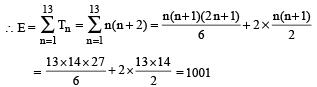= 7 × 11 × 13
Hence the sum of the prime factors of E
= 7 + 11 + 13 = 31.

QUESTION: 4

Arrange the numbers 27/6, 33/4 and 52/3 in ascending order.

(2013)

Solution:

LCM of 6, 4 and 3 = 12
Multiply by 12 of each number in power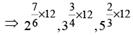⇒ 214, 39, 58
So, ascending order is
58 > 39 > 214  or 52/3 > 33/4 >27/6

QUESTION: 5

The number 44 is written as a product of 5 distinct integers. If ‘n’ is the sum of these five integers then what is the sum of all the possible values of n?

(2012)

Solution:

Prime factorization of 44 is = 2 × 2 × 11
To express 44 as product of five distinct integers
So, we'll have to put 1 and –1.
The only possible way comes out to be:
44 = 2 × (–2) × 11 × 1 × (–1)
In this case the value of n would be 11 which is also the only possible value.

QUESTION: 6

500! + 505! + 510! + 515! is completely divisible by 5n, where n is a natural number. How many distinct values of n are possible?

(2012)

Solution:

500! + 505! + 510! + 515!
= 500! (1 + 5k) (where k is a natural number)
So (5k + 1) won't be a multiple of 5.
Minimum value of n for which 500 ! is divisible by 5n = 1.
Maximum value of n for which 500! is divisible by 5n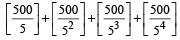= 100 + 20 + 4 = 124
Hence, there are 124 possible values of n.

QUESTION: 7

A sequence of terms is defined such that 2an = an+1 + an-1 ; a0 = 1; a= 3 What is the value of a0 + a1 + a2 + 3 +......+ a50?

(2012)

Solution:

Sum of a0 + a1 + .... + a50 = 1 + 3 + .... + 101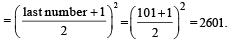QUESTION: 8

There are five consecutive integers a, b, c, d and e such that a < b < c < d < e and a2 + b2 + c2 = d2 + e2. What is/are the possible value(s) of b?

(2012)

Solution:

Let first integer = (x – 1), then
Second integer = x; so..... on
According to question.
⇒ (x  – 1)2 + x2 + (x + 1)2 = (x + 2)2 + (x + 3)2
⇒ x2 + 1 – 2x + x2 + x2 + 1 + 2x = x2 + 4 + 2.x.2 + x2 + 9 + 2.x.3
⇒ 3x2 + 2 = x2 + 4 + 4x + x2 + 9 + 6x
⇒ 3x2 + 2 = 2x2 + 10x + 13
⇒ 3x2 + 2 – 2x2  – 10x – 13 = 0
⇒ x2 – 10x – 11 = 0
⇒ x2 – 11x + x – 11 = 0
⇒ x (x – 11) + 1 (x – 11) = 0
⇒ (x + 1) (x – 11)
∴ x = –1 or 11

QUESTION: 9

All the two-digit natural numbers whose unit digit is greater than their ten’s digit are selected. If all these numbers are written one after the other in a series, how many digits are there in the resulting number?

(2012)

Solution:

Here find the number of two–digit natural numbers such that unit digit is greater than their ten's digit.
In such natural numbers, we cannot take 0 or 1 in units place.
When we take 2 at unit's place, we obtain only 1 Such number is 12.
When we take 3 at unit's place, we obtain 2 such numbes are 13 and 23.
When we take 9 at unit's place, we obtain 8 such numbers.
So, number of such numbers is (1 + 2 + 3 + .... + 8) = 36
Hence, the required number has 72 digits.

QUESTION: 10

(x – 1)(x – 2)(x – 3) = 6y. How many integer solutions exist for the given equation?

(2011)

Solution:

In the given equation the right hand side contains the powers of 2 and 3 only; therefore the left hand side should contain the powers of 2 and 3 only.
Since (x – 1)(x – 2)(x – 3) is a product of three consecutive numbers, it will always contain either one or two multiples of 2 and one multiple of 3. Lets make two cases:
(1) If (x – 1) and (x – 3) are multiples of 2:
Let (x – 1) be equal to 2k; then (x – 3) is equal to 2(k + 1).
Now k and (k + 1) should both contain powers of 2 or 3 only. This is possible with k = 1, 2 or 3. Also if any of k or (k + 1) is a multiple of 3, (x – 2) will not be a multiple of 3 or 2. So again it will not satisfy.
(2) If (x – 2) is a multiple of 2:
Here (x – 1) and (x – 3) will both be odd, out of which only one will be a multiple of 3. Hence the other number will be a multiple of an odd number other than 3. So the equation can be satisfied only if that other odd number is 1. Hence taking one odd number as 1 we get 1 × 2 × 3 which is equal to 6.
Hence the equation is satisfied for x = 4 only.

QUESTION: 11

A positive integer is equal to the square of the number of factors it has. How many such integers are there?

(2011)

Solution:

One such number is 1 which has no factor other than itself.
If the number has only one prime factor i.e. it is of the form pa where p is a prime number and a is a natural number, then according to the question: (a + 1)2 = pa
This is possible only if a = 2 and p = 3. So the number is 9.
If the number has two prime factors then it would be of the type pa × qb, where p and q are two distinct prime numbers. Then according to the question:
(a + 1)2 (b + 1)2 = pa × qb
This is possible only if p and q are both 3. Since they are different, this is not a valid case. So there would no such case with two or more prime factors.
So there are only two such integers - 1 and 9.

QUESTION: 12

(X + 3)/3, (X + 8)/4, (X + 15)/5, (X + 24)/6 ... ((X + 80)/10  is a sequence where X ≠ 1
What is the least value of X for which HCF (Numerator, Denominator) = 1 for each term of the given sequence?

(2011)

Solution:

The general term is of the form
(X + n(n + 2)) / (n + 2)
n(n + 2) is always divisible by (n + 2).
So we can say that n(n + 2) ± 1 would never be divisible by (n + 2).
If we put X = –1, the numerator and denominator of all the terms would be co-prime.

QUESTION: 13

Let S denote the infinite sum 2 + 5x + 9x2 +14x3 + 20x4 + ....... , where | x | < 1 and the coefficient of xn-1 is 1/2 n(n + 3), (n = 1,2,.....) Then S equals

(2010)

Solution:

From option (a),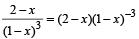Using Binomial here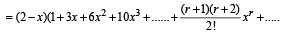= 2 + 5 x + 9x2 + 14x+ .....
this is same series as given
Thus, option (a) is correct answer.

QUESTION: 14

Mr. Mehra is planning for higher education expenses of his two sons aged 15 and 12. He plans to divide ₹ 15 lakhs in two equal parts and invest in two different plans such that his sons may have access to ₹ 21 lakhs each when they reach the age of 21. He is looking for plan that will give him a simple interest per annum. The rates of interest of the plans for his younger son and elder son should be

(2010)

Solution:

For the younger child ₹ 7.5 lakh should become 21 lakhs in 9 years.
Hence,
Amount = Principal + Simple Interest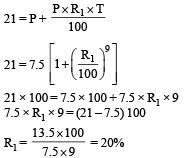Similarly, for the elder son, ₹ 7.5 lakh should become in 6 years.
Hence,  Amount = Principal + Simple Interest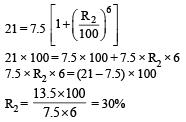QUESTION: 15

The last digit of 334n + 1, is

(2010)

Solution:

Consider 34n = (81)n = (1 + 80)n = 1 + 80q, q ∈ N
∴ 334n = 380q + 1 = (81)20q.3
Since the last digit of (81)20q is 1, so the last digit of
334n + 1 is 1 × 3 + 1 = 4

QUESTION: 16

What is the number of non-negative integer solutions for the equation x2 – xy + y2 = x + y?

(2010)

Solution:

(x2 – xy + y2) = (x + y)
Multiplying both sides by 2:
2(x2– xy + y2) = 2(x + y) ⇒ 2x2 – 2xy + 2y2 = 2(x + y)
(x – y)2 + x2 + y2 = 2x + 2y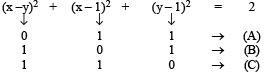Integer solutions for (x, y):
Case 1: (0, 0) and (2, 2)
Case 2: (1, 2) and (1, 0)
Case 3: (2, 1) and (0, 1)
So there are six non-negative integer solutions.

QUESTION: 17

If ‘a’ is one of the roots of x5 – 1 = 0 and a ≠ 1, then what is the value of a15 + a16 + a17 +.......a50?

(2010)

Solution:

a15 + a16 + a17 + .... + a50|
Sum = a15 {1 + a + a2  + ..... a35}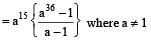Since a is the root of equation x5 – 1 = 0,
a5 – 1 = 0 ⇒ a5 = 1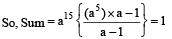QUESTION: 18

If x and y are positive integers, then the last digit of which of the following is same as the last digit of the sum of x and y?

(2010)

Solution:

The cyclicity of each digit from 0 to 9 is a factor of 4.
Hence any digit raised to a power of the type 4k + 1 will always end in the same digit. Hence the answer is x13 + y13.

QUESTION: 19

If ‘n’ is a natural number then the greatest integer less than or equal to (5 + √19)n

(2010)

Solution:

Putting n = 1, we get 5 + √19 whose integral part is 9.
Putting n = 2, we get 25 + 19 + 10√19 whose integral part is 25 + 19 + 43 which is again an odd number.
Now, through the options it can be judged that the greatest integer must always be an odd number.

QUESTION: 20

P, Q and R are three consecutive odd numbers in ascending order. If the value of three times P is three less than two times R, find the value of R.

(2009)

Solution:

Let P, Q and R be n, n + 2 and n + 4 respectively in ascending order.
According to the Question
3n = 2(n + 4) – 3 = 2n + 5
∴ n = 5
Thus, R = 5 + 4 = 9